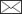Simo Poso, Mark-Leo Waite

Calculation and comparison of different permanent sample plot types .

Poso S., Waite M.-L. (1995). Calculation and comparison of different permanent sample plot types . Silva Fennica vol. 29 no. 2 article id 5554. https://doi.org/10.14214/sf.a9205

Abstract

A calculation procedure is presented for calculating and analysing remeasured permanent sample plots. Data for eight different fixed and variable size plot types were simulated on the basis of two stands whose trees were mapped and measured in 1982 and 1986. The accuracy and efficiency of the plot types were assessed and compared.

The calculation procedure is based on tree-wise expansion factors and the division of tree sampled into state/measurement classes. Nine classes were required for variable size plots and six for fixed size plots. A relascope plot with basal-area factor 1 (m2/ha) proved to be most efficient for estimating basal-area at a given time and a fixed size circular plot with radius 10 m for estimating basal-area increment over a given time period.

The main problems were related to the estimation of non-measurable variables, e.g., the initial diameters of ingrowth trees, i.e., trees having passed the threshold size during the measurement period. Most problematic were cut trees belonging to the ingrowth or sample enlargement classes. It is nevertheless thought that the system is appropriate for monitoring forest changes and making sensitivity analyses with permanent sample plots.

Published in 1995

Views 204

Available at https://doi.org/10.14214/sf.a9205 |Register
Click this link to register to Silva Fennica.